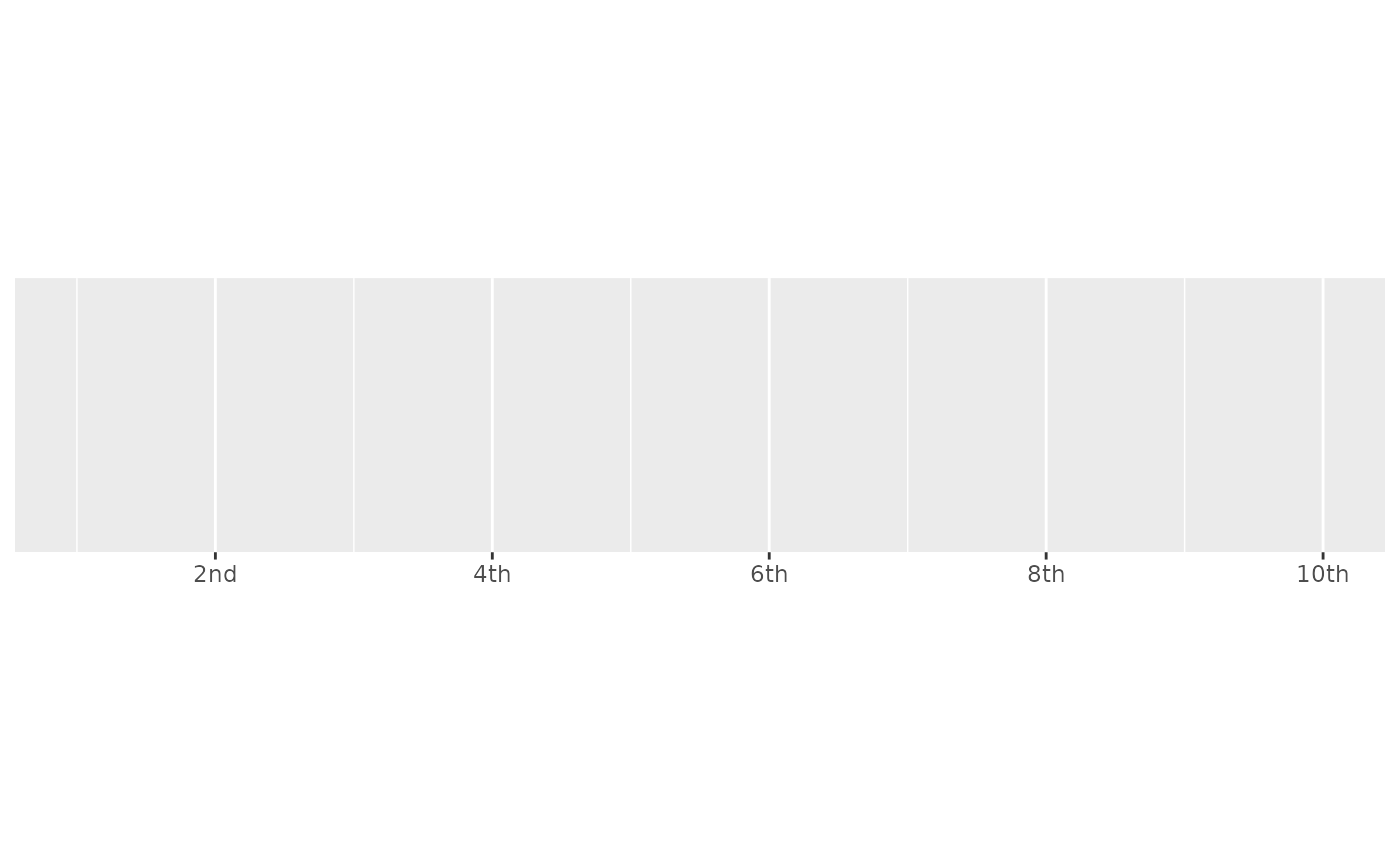Round values to integers and then display as ordinal values (e.g. 1st, 2nd, 3rd). Built-in rules are provided for English, French, and Spanish.

label_ordinal(
prefix = "",
suffix = "",
big.mark = " ",
rules = ordinal_english(),
...
)

ordinal_english()

ordinal_french(gender = c("masculin", "feminin"), plural = FALSE)

ordinal_spanish()

ordinal_format(
prefix = "",
suffix = "",
big.mark = " ",
rules = ordinal_english(),
...
)

ordinal(
x,
prefix = "",
suffix = "",
big.mark = " ",
rules = ordinal_english(),
...
)

## Arguments

prefix, suffix Symbols to display before and after value. Character used between every 3 digits to separate thousands. Named list of regular expressions, matched in order. Name gives suffix, and value specifies which numbers to match. Other arguments passed on to base::format(). Masculin or feminin gender for French ordinal. Plural or singular for French ordinal. A numeric vector to format.

## Value

All label_() functions return a "labelling" function, i.e. a function that takes a vector x and returns a character vector of length(x) giving a label for each input value.

Labelling functions are designed to be used with the labels argument of ggplot2 scales. The examples demonstrate their use with x scales, but they work similarly for all scales, including those that generate legends rather than axes.

## Old interface

ordinal() and format_ordinal() are retired; please use label_ordinal() instead.

## See also

Other labels for continuous scales: label_bytes(), label_dollar(), label_number_auto(), label_number_si(), label_parse(), label_percent(), label_pvalue(), label_scientific()

## Examples

demo_continuous(c(1, 5))
#> scale_x_continuous()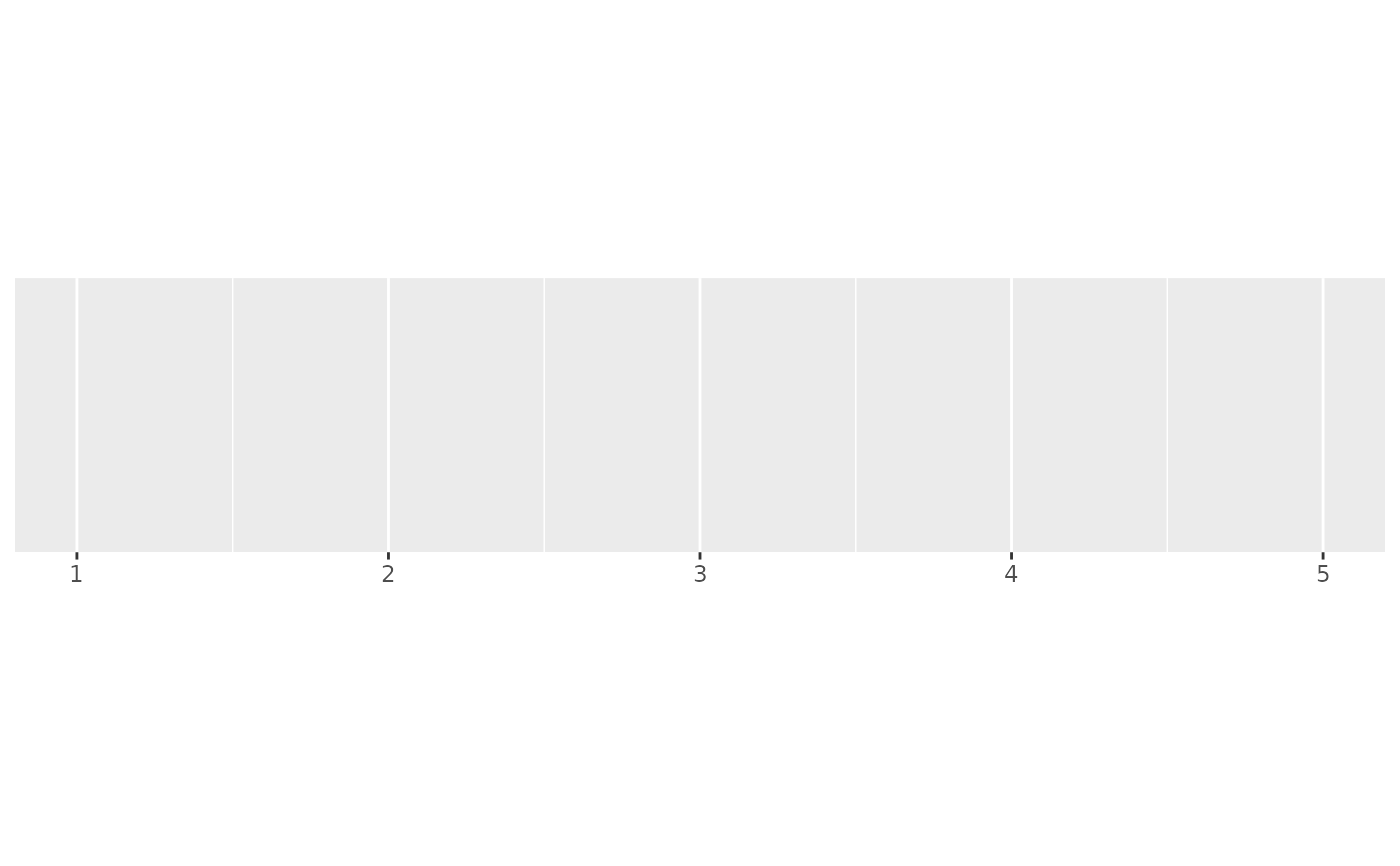demo_continuous(c(1, 5), labels = label_ordinal())
#> scale_x_continuous(labels = label_ordinal())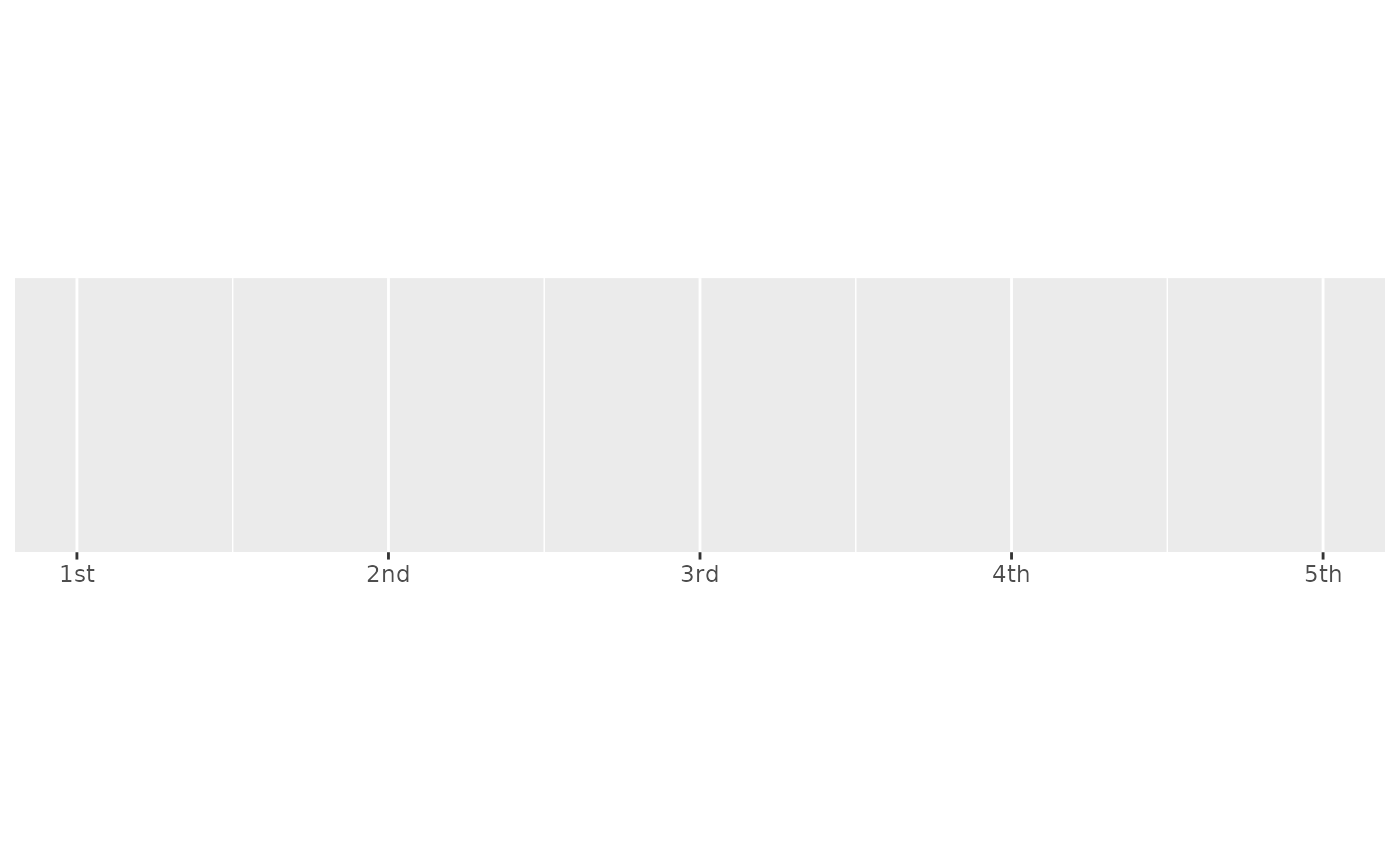demo_continuous(c(1, 5), labels = label_ordinal(rules = ordinal_french()))
#> scale_x_continuous(labels = label_ordinal(rules = ordinal_french()))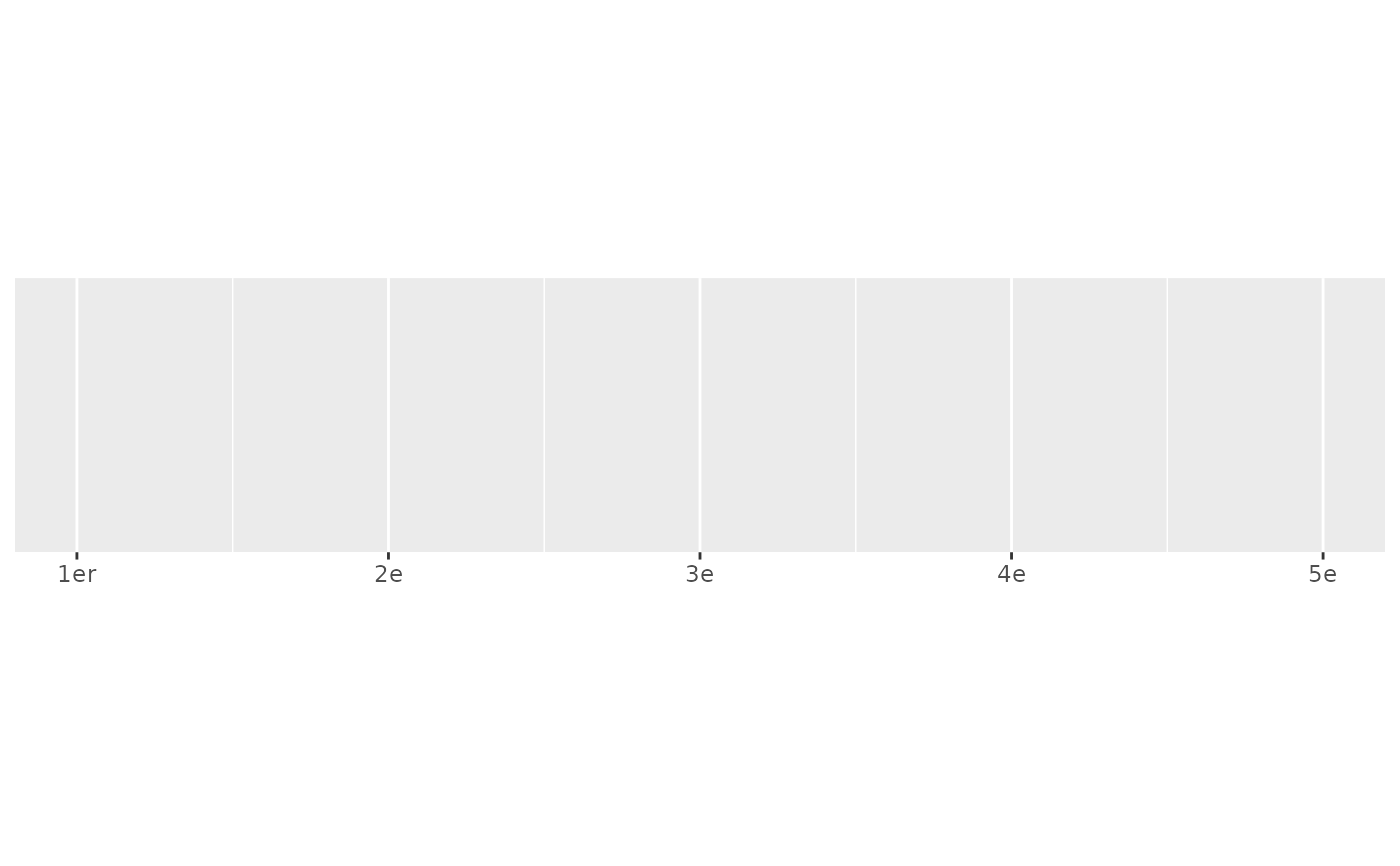# The rules are just a set of regular expressions that are applied in turn
ordinal_french()
#> $er #>  "^1$"
#>
#> $e #>  "." #> ordinal_english() #>$st
#>  "(?<!1)1$" #> #>$nd
#>  "(?<!1)2$" #> #>$rd
#>  "(?<!1)3$" #> #>$th
#>  "(?<=1)$" #> #>$th
#>  "$" #> #>$th
#>  "."
#>
# Note that ordinal rounds values, so you may need to adjust the breaks too
demo_continuous(c(1, 10))
#> scale_x_continuous()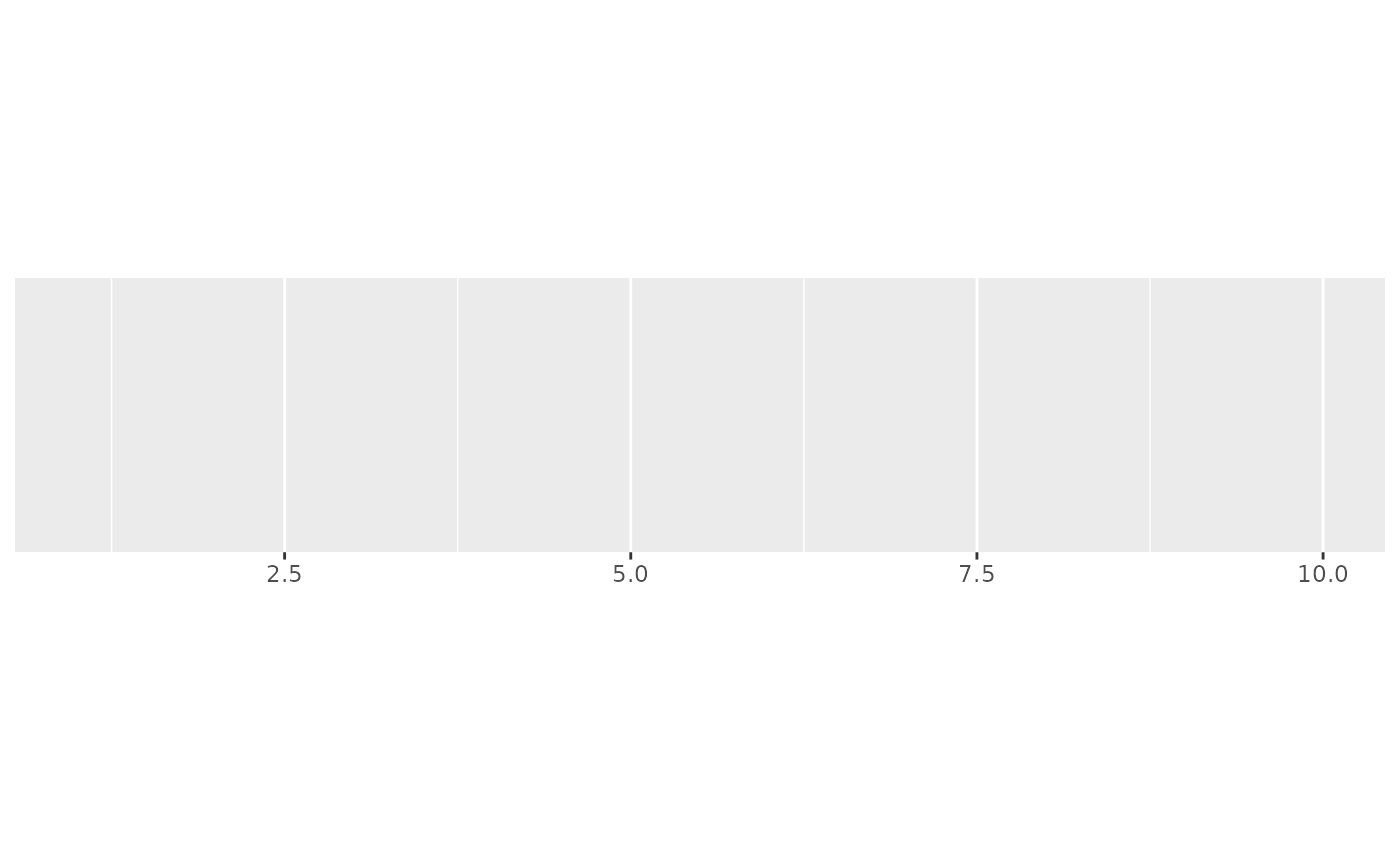demo_continuous(c(1, 10), labels = label_ordinal())
#> scale_x_continuous(labels = label_ordinal())demo_continuous(c(1, 10),
labels = label_ordinal(),
breaks = breaks_width(2)
)
#> scale_x_continuous(labels = label_ordinal(), breaks = breaks_width(2))